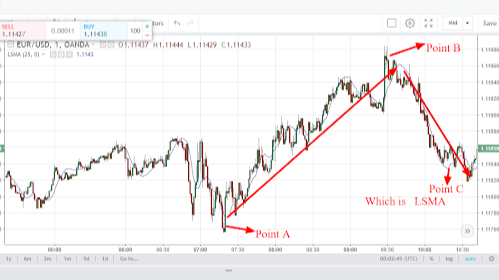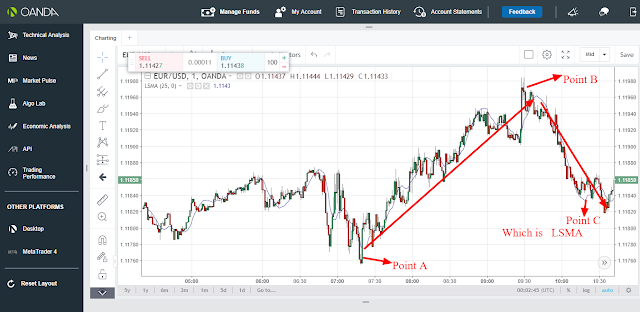# Least Square Moving Average:How to analyze the direction of the financial market using LSMA

Least square Moving Average is a trend following indicator.

Being a trend following indicator,Least square Moving Average indicator was created with the main objective of helping traders to know whether the market is in an upward direction or a downward direction.Least Square Moving Average is also called Linear regression moving average.Just like in other trend following indicator,least square moving average is also based on support and resistance.

The values of Least square moving average is therefore calculated using the following formula;

LSMA= h*t+b  where as

### b=1/nΣxi-h*1/nΣti

while t is the time and n is the period

Since least square moving average is a trend following indicator and is based on support and resistance, it therefore follows that when the market is in an uptrend movement,the least square moving average will also be moving upwards while when the market is in a downward trend, the least square moving average will also be moving downwards.Based on support and resistance, when the price rises above the least square moving average  when the two are in an uptrend movement, a downward reversal movement will take place and the two will start moving downwards thus signaling the trader to be trading downwards.On the other hand, when the price falls below the least square moving average  when the market and the least square moving average are in a downwards trend, an upward market reversal will take place and the two will now be moving downwards thus signaling the trader to be trading upwards.This is indicated as from the candle sticks chart below;From the candle sticks chart above, there are 3 points, point A, B and C. Point A and B represent the support and resistance point while point C which is the blue line is the least square moving average.At point A, the least square moving average and the market were initially moving downwards.The price then falls below the least square moving average thus resulting to an upward reversal for both the least square moving average and the market thus signaling the trader to close any sell position and open a buy position since the movement was now upward as indicated by the upward red arrow.On the other hand,at point B, the least square moving average and the market were initially moving upwards.The price then rises above the least square moving average thus resulting to a downward reversal movement for both the least square moving average and the market thus signaling the trader to close any buy position and open a sell position since the movement was now downwards.

### Recommendation:If you are a day trader just use 1 min,5 min, 15 min and 30 min timeframe while if you are a swing trader just use 1 hour and above timeframe if you want least square moving average to work well for you.

You are always free to support our work from below;

skrill donation:[email protected]

Neteller donation:[email protected]

Bitcoin:3AFeiwUp6erj2iRBmdsnc7YhC7gcYVD6oj

Ethereum; 0x346570c491b76c7cd51699bdd272762111b743a9

Litecoin: LeEH7B82ccXJ6QoibCryGJNpDGNx2x77fL

Dogecoin: DLShs2vqhMc1ggqo8MPPMBTcPAZdqCZoWd

lightning network:tipping.me:https://tippin.me/@quintomudigoTrader, Blockchain Technologist and Contentpreneur. Also founder and CEO @ Quinto Trader